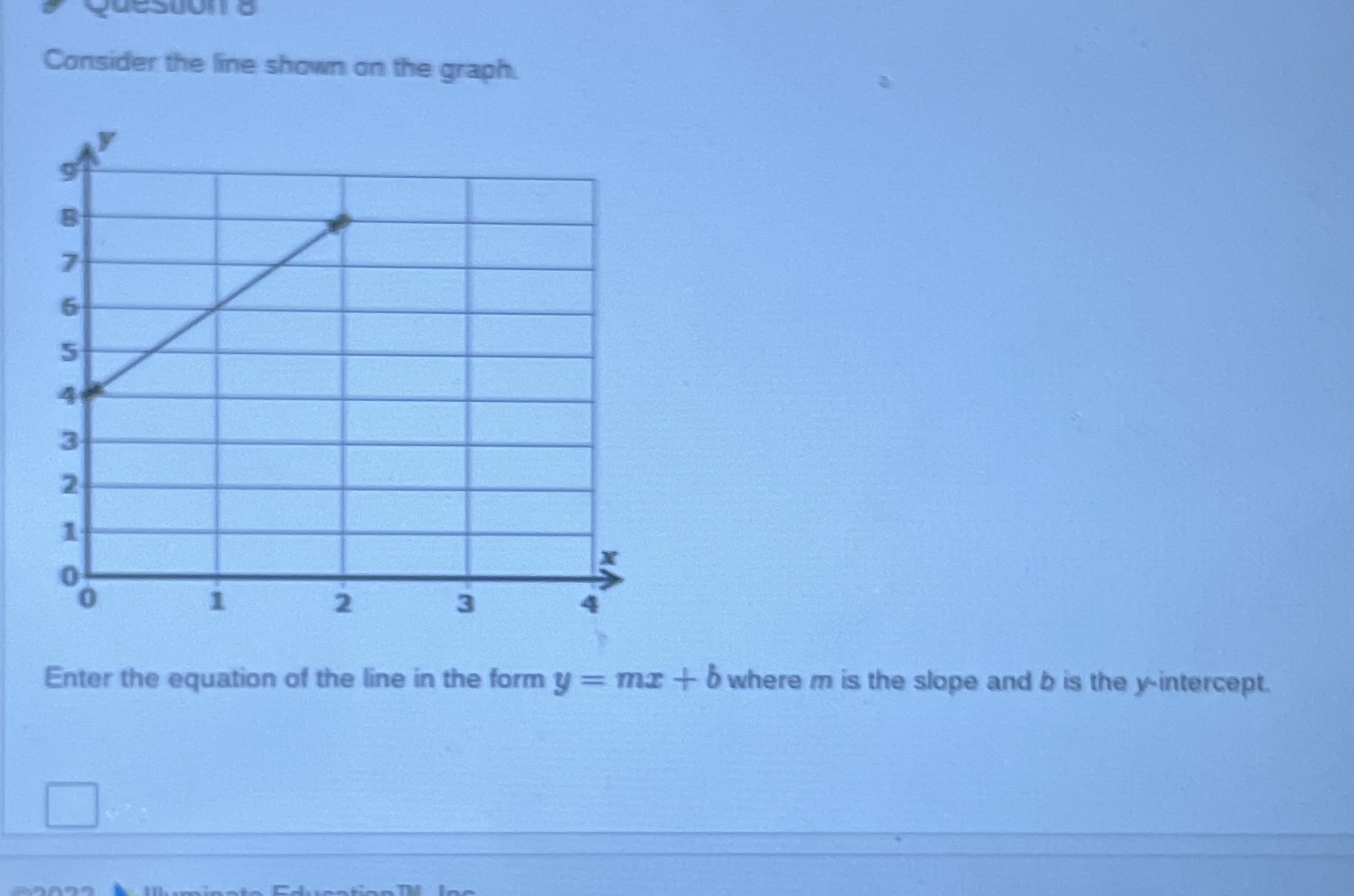### ¿Todavía tienes preguntas de matemáticas?

Pregunte a nuestros tutores expertos
Algebra
PreguntaConsider the line shown on the graph. Enter the equation of the line in the form $$y = m x + b$$ where $$m$$ is the slope and $$b$$ is the $$y$$ -intercept.